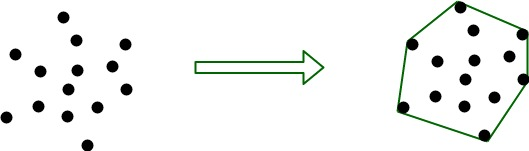Related Articles
Quickhull Algorithm for Convex Hull
• Difficulty Level : Hard
• Last Updated : 11 Oct, 2019

Given a set of points, a Convex hull is the smallest convex polygon containing all the given points.Input is an array of points specified by their x and y coordinates. Output is a convex hull of this set of points in ascending order of x coordinates.

Example :

```Input : points[] = {{0, 3}, {1, 1}, {2, 2}, {4, 4},
{0, 0}, {1, 2}, {3, 1}, {3, 3}};
Output :  The points in convex hull are:
(0, 0) (0, 3) (3, 1) (4, 4)

Input : points[] = {{0, 3}, {1, 1}
Output : Not Possible
There must be at least three points to form a hull.

Input  : points[] = {(0, 0), (0, 4), (-4, 0), (5, 0),
(0, -6), (1, 0)};
Output : (-4, 0), (5, 0), (0, -6), (0, 4)
```

## Recommended: Please solve it on “PRACTICE” first, before moving on to the solution.

We have discussed following algorithms for Convex Hull problem.
Convex Hull | Set 1 (Jarvis’s Algorithm or Wrapping)
Convex Hull | Set 2 (Graham Scan)

The QuickHull algorithm is a Divide and Conquer algorithm similar to QuickSort. Let a[0…n-1] be the input array of points. Following are the steps for finding the convex hull of these points.

1. Find the point with minimum x-coordinate lets say, min_x and similarly the point with maximum x-coordinate, max_x.
2. Make a line joining these two points, say L. This line will divide the whole set into two parts. Take both the parts one by one and proceed further.
3. For a part, find the point P with maximum distance from the line L. P forms a triangle with the points min_x, max_x. It is clear that the points residing inside this triangle can never be the part of convex hull.
4. The above step divides the problem into two sub-problems (solved recursively). Now the line joining the points P and min_x and the line joining the points P and max_x are new lines and the points residing outside the triangle is the set of points. Repeat point no. 3 till there no point left with the line. Add the end points of this point to the convex hull.

Below is C++ implementation of above idea. The implementation uses set to store points so that points can be printed in sorted order. A point is represented as a pair.

 `// C++ program to implement Quick Hull algorithm``// to find convex hull.``#include``using` `namespace` `std;`` ` `// iPair is integer pairs``#define iPair pair`` ` `// Stores the result (points of convex hull)``set hull;`` ` `// Returns the side of point p with respect to line``// joining points p1 and p2.``int` `findSide(iPair p1, iPair p2, iPair p)``{``    ``int` `val = (p.second - p1.second) * (p2.first - p1.first) -``              ``(p2.second - p1.second) * (p.first - p1.first);`` ` `    ``if` `(val > 0)``        ``return` `1;``    ``if` `(val < 0)``        ``return` `-1;``    ``return` `0;``}`` ` `// returns a value proportional to the distance``// between the point p and the line joining the``// points p1 and p2``int` `lineDist(iPair p1, iPair p2, iPair p)``{``    ``return` `abs` `((p.second - p1.second) * (p2.first - p1.first) -``               ``(p2.second - p1.second) * (p.first - p1.first));``}`` ` `// End points of line L are p1 and p2.  side can have value``// 1 or -1 specifying each of the parts made by the line L``void` `quickHull(iPair a[], ``int` `n, iPair p1, iPair p2, ``int` `side)``{``    ``int` `ind = -1;``    ``int` `max_dist = 0;`` ` `    ``// finding the point with maximum distance``    ``// from L and also on the specified side of L.``    ``for` `(``int` `i=0; i max_dist)``        ``{``            ``ind = i;``            ``max_dist = temp;``        ``}``    ``}`` ` `    ``// If no point is found, add the end points``    ``// of L to the convex hull.``    ``if` `(ind == -1)``    ``{``        ``hull.insert(p1);``        ``hull.insert(p2);``        ``return``;``    ``}`` ` `    ``// Recur for the two parts divided by a[ind]``    ``quickHull(a, n, a[ind], p1, -findSide(a[ind], p1, p2));``    ``quickHull(a, n, a[ind], p2, -findSide(a[ind], p2, p1));``}`` ` `void` `printHull(iPair a[], ``int` `n)``{``    ``// a[i].second -> y-coordinate of the ith point``    ``if` `(n < 3)``    ``{``        ``cout << ``"Convex hull not possible\n"``;``        ``return``;``    ``}`` ` `    ``// Finding the point with minimum and``    ``// maximum x-coordinate``    ``int` `min_x = 0, max_x = 0;``    ``for` `(``int` `i=1; i a[max_x].first)``            ``max_x = i;``    ``}`` ` `    ``// Recursively find convex hull points on``    ``// one side of line joining a[min_x] and``    ``// a[max_x]``    ``quickHull(a, n, a[min_x], a[max_x], 1);`` ` `    ``// Recursively find convex hull points on``    ``// other side of line joining a[min_x] and``    ``// a[max_x]``    ``quickHull(a, n, a[min_x], a[max_x], -1);`` ` `    ``cout << ``"The points in Convex Hull are:\n"``;``    ``while` `(!hull.empty())``    ``{``        ``cout << ``"("` `<<( *hull.begin()).first << ``", "``             ``<< (*hull.begin()).second << ``") "``;``        ``hull.erase(hull.begin());``    ``}``}`` ` `// Driver code``int` `main()``{``    ``iPair a[] = {{0, 3}, {1, 1}, {2, 2}, {4, 4},``               ``{0, 0}, {1, 2}, {3, 1}, {3, 3}};``    ``int` `n = ``sizeof``(a)/``sizeof``(a);``    ``printHull(a, n);``    ``return` `0;``}`

Input :

```The points in Convex Hull are:
(0, 0) (0, 3) (3, 1) (4, 4)
```

Time Complexity: The analysis is similar to Quick Sort. On average, we get time complexity as O(n Log n), but in worst case, it can become O(n2)

This article is contributed by Amritya Yagni. If you like GeeksforGeeks and would like to contribute, you can also write an article using contribute.geeksforgeeks.org or mail your article to contribute@geeksforgeeks.org. See your article appearing on the GeeksforGeeks main page and help other Geeks.

Please write comments if you find anything incorrect, or you want to share more information about the topic discussed above.

Attention reader! Don’t stop learning now. Get hold of all the important DSA concepts with the DSA Self Paced Course at a student-friendly price and become industry ready.

My Personal Notes arrow_drop_up